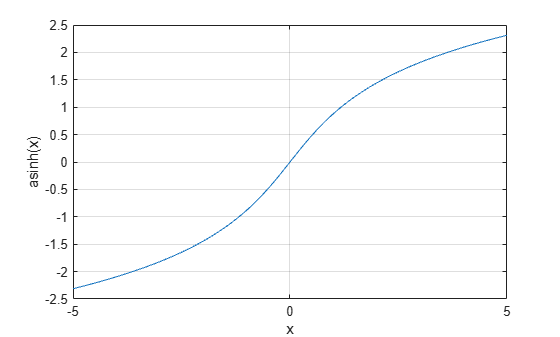# asinh

Inverse hyperbolic sine

## Syntax

``Y = asinh(X)``

## Description

example

````Y = asinh(X)` returns the inverse hyperbolic sine of the elements of `X`. The function accepts both real and complex inputs. All angles are in radians.```

## Examples

collapse all

Find the inverse hyperbolic sine of the elements of vector `X`. The `asinh` function acts on `X` element-wise.

```X = [2 -3 1+2i]; Y = asinh(X)```
```Y = 1×3 complex 1.4436 + 0.0000i -1.8184 + 0.0000i 1.4694 + 1.0634i ```

Plot the inverse hyperbolic sine function over the interval $-5\le x\le 5$.

```x = -5:.01:5; plot(x,asinh(x)) grid on xlabel('x') ylabel('asinh(x)')```## Input Arguments

collapse all

Hyperbolic sine of angle, specified as a scalar, vector, matrix, or multidimensional array. The `asinh` operation is element-wise when `X` is nonscalar.

Data Types: `single` | `double`
Complex Number Support: Yes

collapse all

### Inverse Hyperbolic Sine

For real values $x$ in the domain of all real numbers, the inverse hyperbolic sine satisfies

`${\mathrm{sinh}}^{-1}\left(x\right)=\mathrm{log}\left(x+\sqrt{{x}^{2}+1}\right).$`

For complex numbers $z=x+iy$, the call `asinh(z)` returns complex results.

## Version History

Introduced before R2006a